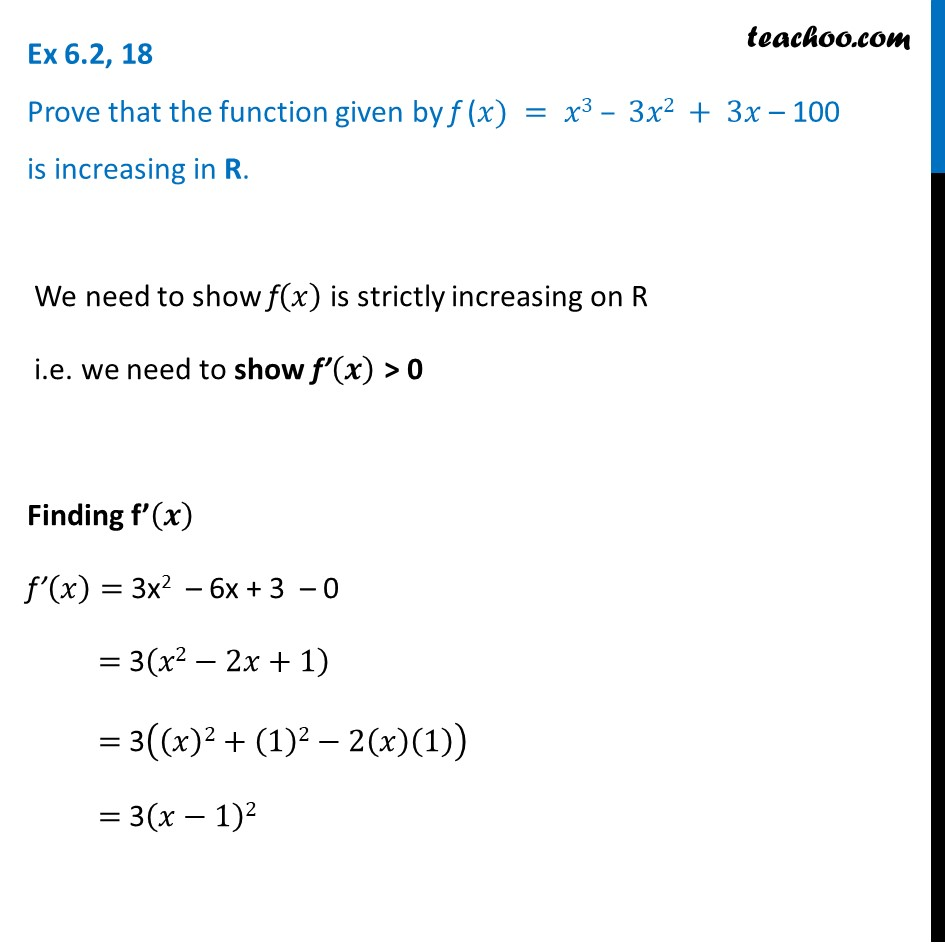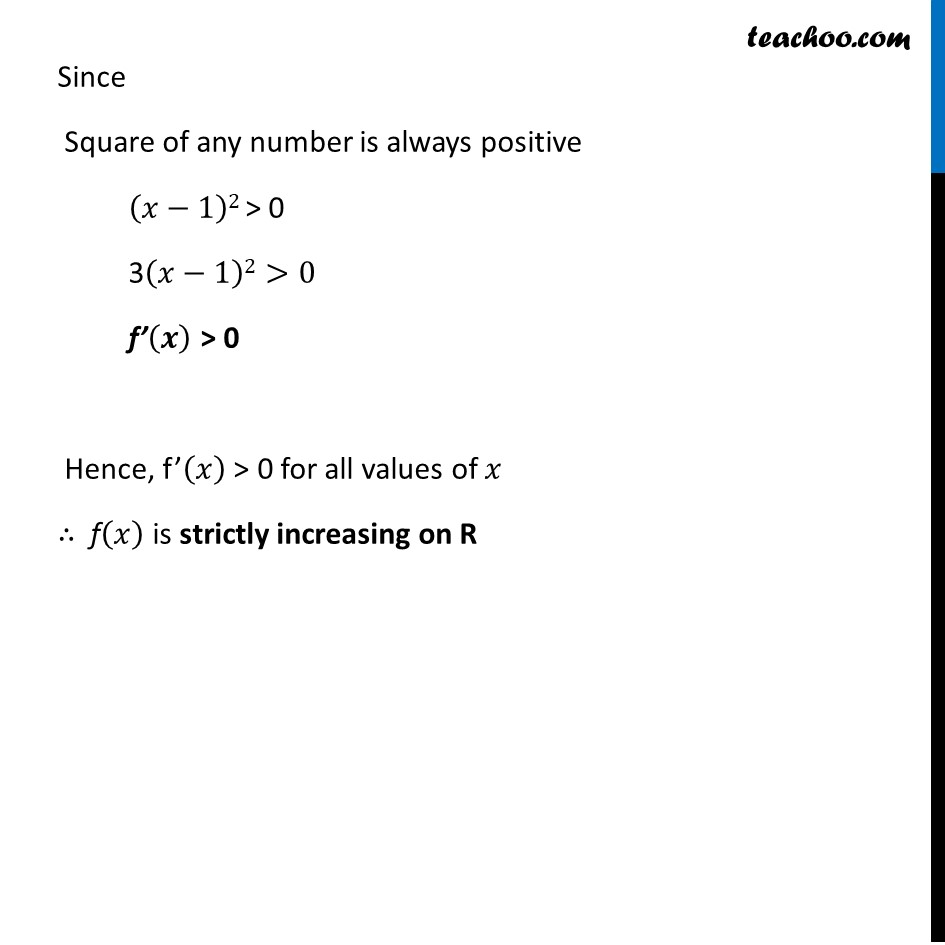1. Chapter 6 Class 12 Application of Derivatives (Term 1)
2. Serial order wise
3. Ex 6.2

Transcript

Ex 6.2, 18 Prove that the function given by f (𝑥) = 𝑥3 – 3𝑥2 + 3𝑥 – 100 is increasing in R. We need to show f(𝑥) is strictly increasing on R i.e. we need to show f’(𝒙) > 0 Finding f’(𝒙) f’(𝑥)= 3x2 – 6x + 3 – 0 = 3(𝑥2−2𝑥+1) = 3((𝑥)2+(1)2−2(𝑥)(1)) = 3(𝑥−1)2 Since Square of any number is always positive (𝑥−1)2 > 0 3(𝑥−1)2>0 f’(𝒙) > 0 Hence, f’(𝑥) > 0 for all values of 𝑥 ∴ f(𝑥) is strictly increasing on R

Ex 6.2

Chapter 6 Class 12 Application of Derivatives (Term 1)
Serial order wise